Next: Electromagnetic Waves Up: Electromagnetic Waves Previous: Electromagnetic Waves

## Maxwell's Equations

In the latter half of the nineteenth century, the Scottish physicist James Clerk Maxwell demonstrated that all previously established experimental facts regarding electric and magnetic fields could be summed up in just four equations. Nowadays, these equations are generally known as Maxwell's equations.

The first equation is simply Gauss' law (see Sect. 4). This equation describes how electric charges generate electric fields. Gauss' law states that:

The electric flux through any closed surface is equal to the total charge enclosed by the surface, divided by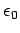.
This can be written mathematically as(305)

whereis a closed surface enclosing the charge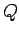. The above expression can also be written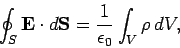(306)

whereis a volume bounded by the surface, and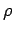is the charge density: i.e., the electric charge per unit volume.

The second equation is the magnetic equivalent of Gauss' law (see Sect. 8.10). This equation describes how the non-existence of magnetic monopoles causes magnetic field-lines to form closed loops. Gauss' law for magnetic fields states that:

The magnetic flux through any closed surface is equal to zero.
This can be written mathematically as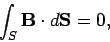(307)

whereis a closed surface.

The third equation is Faraday's law (see Sect. 9.3). This equation describes how changing magnetic fields generate electric fields. Faraday's law states that:

The line integral of the electric field around any closed loop is equal to minus the time rate of change of the magnetic flux through the loop.
This can be written mathematically as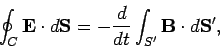(308)

where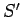is a surface attached to the loop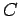.

The fourth, and final, equation is Ampère's circuital law (see Sect. 8.7). This equation describes how electric currents generates magnetic fields. Ampère's circuital law states that:

The line integral of the magnetic field around any closed loop is equal to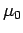times the algebraic sum of the currents which pass through the loop.
This can be written mathematically as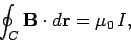(309)

whereis the net current flowing through loop. This equation can also be written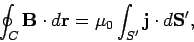(310)

whereis a surface attached to the loop, and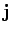is the current density: i.e., the electrical current per unit area.

When Maxwell first wrote Eqs. (306), (307), (308), and (310) he was basically trying to summarize everything which was known at the time about electric and magnetic fields in mathematical form. However, the more Maxwell looked at his equations, the more convinced he became that they were incomplete. Eventually, he proposed adding a new term, called the displacement current, to the right-hand side of his fourth equation. In fact, Maxwell was able to show that (306), (307), (308), and (310) are mathematically inconsistent unless the displacement current term is added to Eq. (310). Unfortunately, Maxwell's demonstration of this fact requires some advanced mathematical techniques which lie well beyond the scope of this course. In the following, we shall give a highly simplified version of his derivation of the missing term.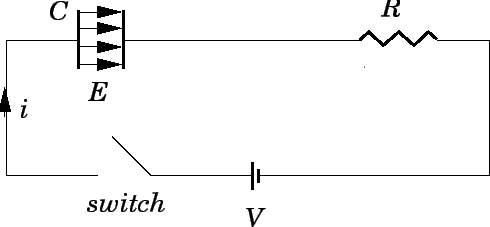Consider a circuit consisting of a parallel plate capacitor of capacitancein series with a resistanceand an steady emf, as shown in Fig. 52. Letbe the area of the capacitor plates, and letbe their separation. Suppose that the switch is closed at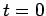. The current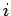flowing around the circuit starts from an initial value of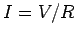, and gradually decays to zero on the RC time of the circuit (see Sect. 10.5). Simultaneously, the chargeon the positive plates of the capacitor starts from zero, and gradually increases to a final value of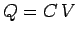. As the chargevaries, so does the potential difference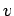between the capacitor plates, since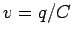.

The electric field in the region between the plates is approximately uniform, directed perpendicular to the plates (running from the positively charged plate to the negatively charged plate), and is of magnitude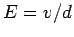. It follows that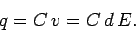(311)

In a time interval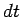, the charge on the positive plate of the capacitor increases by an amount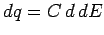, where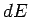is the corresponding increase in the electric field-strength between the plates. Note that bothandare time-independent quantities. It follows that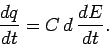(312)

Now,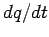is numerically equal to the instantaneous currentflowing around the circuit (since all of the charge which flows around the circuit must accumulate on the plates of the capacitor). Also,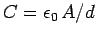for a parallel plate capacitor. Hence, we can write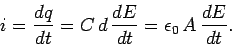(313)

Since the electric fieldis normal to the area, we can also write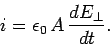(314)

Equation (314) relates the instantaneous current flowing around the circuit to the time rate of change of the electric field between the capacitor plates. According to Eq. (310), the current flowing around the circuit generates a magnetic field. This field circulates around the current carrying wires connecting the various components of the circuit. However, since there is no actual current flowing between the plates of the capacitor, no magnetic field is generated in this region, according to Eq. (310). Maxwell demonstrated that for reasons of mathematical self-consistency there must, in fact, be a magnetic field generated in the region between the plates of the capacitor. Furthermore, this magnetic field must be the same as that which would be generated if the current(i.e., the same current as that which flows around the rest of the circuit) flowed between the plates. Of course, there is no actual current flowing between the plates. However, there is a changing electric field. Maxwell argued that a changing electric field constitutes an effective current (i.e., it generates a magnetic field in just the same manner as an actual current). For historical reasons (which do not particularly interest us at the moment), Maxwell called this type of current a displacement current. Since the displacement current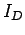flowing between the plates of the capacitor must equal the currentflowing around the rest of the circuit, it follows from Eq. (314) that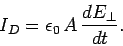(315)

Equation (315) was derived for the special case of the changing electric field generated in the region between the plates of a charging parallel plate capacitor. Nevertheless, this equation turns out to be completely general. Note that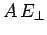is equal to the electric flux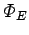between the plates of the capacitor. Thus, the most general expression for the displacement current passing through some closed loop is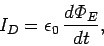(316)

whereis the electric flux through the loop.

According to Maxwell's argument, a displacement current is just as effective at generating a magnetic field as a real current. Thus, we need to modify Ampère's circuital law to take displacement currents into account. The modified law, which is known as the Ampère-Maxwell law, is written

The line integral of the electric field around any closed loop is equal totimes the algebraic sum of the actual currents and which pass through the loop plustimes the displacement current passing through the loop.
This can be written mathematically as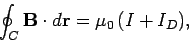(317)

whereis a loop through which the electric currentand the displacement currentpass. This equation can also be written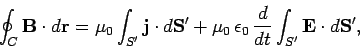(318)

whereis a surface attached to the loop.

Equations (306), (307), (308), and (318) are known collectively as Maxwell's equations. They constitute a complete and mathematically self-consistent description of the behaviour of electric and magnetic fields.Next: Electromagnetic Waves Up: Electromagnetic Waves Previous: Electromagnetic Waves
Richard Fitzpatrick 2007-07-14